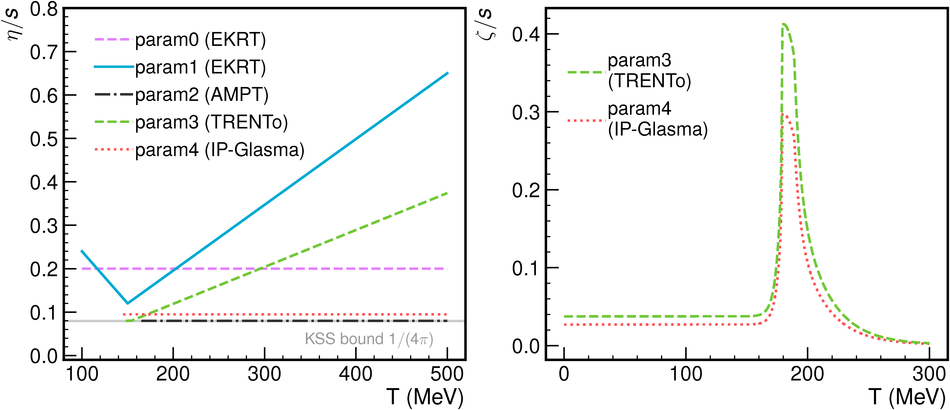# Figure 1

 The five different parameterizations of $\eta/s$ and $\zeta/s$ used for the different hydrodynamic model calculations are shown in the left and right panel. Note that the functional form of $\zeta/s(T)$ is the same for param3 and param4 and taken from Eq.~5 in motivated by Refs.~. For the parameters with \trento{} initial condition, the ones based on identified yields are taken from Table~4 in . The $\zeta/s$ normalization factor used with IP-Glasma (\trento) initial conditions is 0.9 (1.25). The models with $\zeta/s=0$ are not shown on the right.